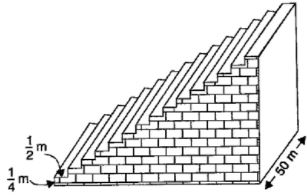# NCERT Solution for Class 10 Mathematics Chapter 5 Arithmetic Progressions

 Chapter Name NCERT Solution for Class 10 Maths Chapter 5 Arithmetic Progressions Topics Covered Short Revision for the ChapterNCERT Exercise Solution Related Study NCERT Solution for Class 10 MathsNCERT Revision Notes for Class 10 MathsImportant Questions for Class 10 MathsMCQ for Class 10 MathsNCERT Exemplar Questions For Class 10 Maths

## Short Revision for Arithmetic Progression

1. A sequence is an arrangement of numbers according to some rule.
2. A sequence may be either a finite or an infinite.
3. A finite sequence has a finite number of terms.
4. An infinite sequence has infinite number of terms.
5. A sequence is called a progression, if its terms are either in an increasing order or a decreasing order.
6. A sequence is called an arithmetic progression if its terms are increasing or decreasing by a constant number.
7. On subtracting any term of an arithmetic progression (AP) from its next term, we get the common difference (d).
8. The common difference of an A.P. can be positive, negative or zero.
9. An A.P. can contain all the terms equal.
10. Generally, we denote first term and common difference of an A.P. by a and d respectively.
11. The general form of an A.P. is a, a + d, a + 2d, a+ 3d, .......
12. To solve problems, if sum of terms of an A.P. is given, then for
(i) 3 terms of an A.P. we take terms as a - d, a, a + d
(ii) 4 terms of an A.P. we take terms as a - 3d, a - d, a + d, a + 3d
(iii) 5 terms of an A.P. we take terms as a - 2d, a - d, a, a + d, a+ 2d.
13. nth term of an A.P. is given by an = a + (n -1)d. This term is also called general term of the A.P.
14. If a,b, c are in A.P. then b – a = c – b or 2b = a +c.
15. If a1 , a2 , a3 , ….. , an is an A.P., then a2 – a1 = a3 – a2 = a4 – a3 = ….. an – an-1 = d.
16. If an is the nth term of an A.P., then its common difference is given by d = an – an-1
where, an-1 is the (n – 1)th term of the A.P.
17. In an A.P. of n terms rth term from the end = (n – r + 1)th term from the beginning.
18. A sequence is an A.P., if an – an-1 is independent of n.
19. A sequence is an A.P., if its nth term is a linear expression in n. In such a case the common difference is the coefficient of n.
20. The sum of first n terms of an A.P. is given by
Sn = n/2 [2a + (n -1)d].
21. If the last term of an A.P. is l and a is the first terms, then the sum of n terms of the A.P. is given by
Sn = n/2(a + 1).
22.  If the last term, say nth term of an A.P. is l, then
l = a + (n -1)d
23. nth term of an A.P., whose sum to n terms, Sn, is given by an = Sn – Sn-1.

### NCERT Exercises Solution

#### Exercise 5.1

1. In which of the following situations, does the list of numbers involved make as arithmetic progression and why?
(i) The taxi fare after each km when the fare is Rs 15 for the first km and Rs 8 for each additional km.
(ii) The amount of air present in a cylinder when a vacuum pump removes 1/4 of the air remaining in the cylinder at a time.
(iii) The cost of digging a well after every metre of digging, when it costs Rs 150 for the first metre and rises by Rs 50 for each subsequent metre.
(iv) The amount of money in the account every year, when Rs 10000 is deposited at compound interest at 8% per annum.

2. Write first four terms of the A.P. when the first term a and the common difference are given as follows:
(i) a = 10, d = 10
(ii) a = -2, d = 0
(iii) a = 4, d = – 3
(iv) a = -1 d = 1/2
(v) a = – 1.25, d = – 0.25

3. For the following A.P.s, write the first term and the common difference.
(i) 3, 1, – 1, – 3 …
(ii) -5, – 1, 3, 7 …
(iii) 1/3, 5/3, 9/3, 13/3 ….
(iv) 0.6, 1.7, 2.8, 3.9 …

4. Which of the following are APs? If they form an A.P. find the common difference d and write three more terms.
(i) 2, 4, 8, 16 …
(ii) 2, 5/2, 3, 7/2 ….
(iii) -1.2, -3.2, -5.2, -7.2 …
(iv) -10, – 6, – 2, 2 …
(v) 3, 3 + √2, 3 + 2√2, 3 + 3√2
(vi) 0.2, 0.22, 0.222, 0.2222 ….
(vii) 0, – 4, – 8, – 12 …
(viii) -1/2, -1/2, -1/2, -1/2 ….
(ix) 1, 3, 9, 27 …
(x) a, 2a, 3a, 4a …
(xi) aa2a3a4 …
(xii) √2, √8, √18, √32 …
(xiii) √3, √6, √9, √12 …
(xiv) 12, 32, 52, 72 …
(xv) 12, 52, 72, 73 …

#### Exercise 5.2

1. Fill in the blanks in the following table, given that a is the first term, d the common difference and an the nth term of the A.P.

 A D N an (i) 7 3 8 …….. (ii) -18 ……. 10 0 (iii) …….. -3 18 -5 (iv) -18.9 2.5 …… 3.6 (v) 3.5 0 105 …….

2. Choose the correct choice in the following and justify:
(i) 30th term of the A.P: 10,7, 4, …, is
(A) 97 (B) 77 (C) −77 (D) −87
(ii) 11th term of the A.P: -3, -1/2, 2, ....... , is
(A) 28 (B) 22  (C) -38  (D)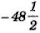4. Which term of the A.P. 3, 8, 13, 18, … is 78?

Solution

Let an  = 78, then 78 = 3 + (n -1)5

⇒ n = 16.

5. Find the number of terms in each of the following A.P.
(i) 7, 13, 19, …, 205
(ii) 18,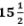, 13, ....., -47

6. Check whether -150 is a term of the A.P. 11, 8, 5, 2, …

7. Find the 31st term of an A.P. whose 11th term is 38 and the 16th term is 73.

8. An AP consists of 50 terms of which 3rd term is 12 and the last term is 106. Find the 29th term.

9. If the 3rd and the 9th terms of an A.P. are 4 and − 8 respectively. Which term of this A.P. is zero.

10. If 17th term of an A.P. exceeds its 10th term by 7. Find the common difference.

11. Which term of the A.P. 3, 15, 27, 39,.. will be 132 more than its 54th term?

12. Two APs have the same common difference. The difference between their 100th term is 100, what is the difference between their 1000th terms?

13. How many three digit numbers are divisible by 7?

Solution

Three - digit numbers divisible by 7 are 105, 112, ......, 994.
Let the required number of numbers be n
∴ 994 = 105 + (n - 1)7 ⇒ 7n = 896 ⇒ n = 128.

14. How many multiples of 4 lie between 10 and 250?

15. For what value of n, are the nth terms of two APs 63, 65, 67, and 3, 10, 17, … equal?

Solution

63 + (n - 1)2 = 3 + (n - 1)7
⇒ 60 = 5(n - 1)

⇒ n = 13.

16. Determine the A.P. whose third term is 16 and the 7th term exceeds the 5th term by 12.

17. Find the 20th term from the last term of the A.P. 3, 8, 13, …, 253.

Solution

From the end, a = 253, d = -5
∴ 20th term from the end = 253 + (20 - 1)(-5)
= 253 - 95 = 158.

18. The sum of 4th and 8th terms of an A.P. is 24 and the sum of the 6th and 10th terms is 44. Find the first three terms of the A.P.

19. Subba Rao started work in 1995 at an annual salary of Rs 5000 and received an increment of Rs 200 each year. In which year did his income reach Rs 7000?

20. Ramkali saved Rs 5 in the first week of a year and then increased her weekly saving by Rs 1.75. If in the nth week, her weekly savings become Rs 20.75, find n.

#### Exercise 5.3

1. Find the sum of the following APs.
(i) 2, 7, 12 ,…., to 10 terms.
(ii) − 37, − 33, − 29 ,…, to 12 terms
(iii) 0.6, 1.7, 2.8 ,…….., to 100 terms
(iv) 1/15, 1/12, 1/10, …… , to 11 terms

2. Find the sums given below :
(i) 7 +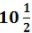+ 14 + ..... + 84
(ii) 34 + 32 +30 + ....... + 10
(iii) -5 + (-8) + (-11) + ...... + (-230)

3. In an AP
(i) Given a = 5, d = 3, an = 50, find n and Sn.
(ii) Given a = 7, a13 = 35, find d and S13.
(iii) Given a12 = 37, d = 3, find a and S12.
(iv) Given a3 = 15, S10 = 125, find d and a10.
(v) Given d = 5, S9 = 75, find a and a9.
(vi) Given a = 2, d = 8, Sn = 90, find n and an.
(vii) Given a = 8, an = 62, Sn = 210, find n and d.
(viii) Given an = 4, d = 2, Sn = − 14, find n and a.
(ix) Given a = 3, n = 8, S = 192, find d.
(x) Given l = 28, S = 144 and there are total 9 terms. Find a.

4. How many terms of the AP. 9, 17, 25 … must be taken to give a sum of 636?

5. The first term of an AP is 5, the last term is 45 and the sum is 400. Find the number of terms and the common difference.

6. The first and the last term of an AP are 17 and 350 respectively. If the common difference is 9, how many terms are there and what is their sum?

7. Find the sum of first 22 terms of an AP in which d = 7 and 22nd term is 149.

8. Find the sum of first 51 terms of an AP whose second and third terms are 14 and 18 respectively.

9. If the sum of first 7 terms of an AP is 49 and that of 17 terms is 289, find the sum of first n terms.

10. Show that a1a… , an , … form an AP where an is defined as below
(i) an = 3+4n
(ii) an = 9−5n
Also find the sum of the first 15 terms in each case.

11. If the sum of the first n terms of an AP is 4n − n2, what is the first term (that is S1)? What is the sum of first two terms? What is the second term? Similarly find the 3rd, the10th and the nth terms.

12. Find the sum of first 40 positive integers divisible by 6.

13. Find the sum of first 15 multiples of 8.

14. Find the sum of the odd numbers between 0 and 50.

15. A contract on construction job specifies a penalty for delay of completion beyond a certain date as follows: Rs. 200 for the first day, Rs. 250 for the second day, Rs. 300 for the third day, etc., the penalty for each succeeding day being Rs. 50 more than for the preceding day. How much money the contractor has to pay as penalty, if he has delayed the work by 30 days.

16. A sum of Rs 700 is to be used to give seven cash prizes to students of a school for their overall academic performance. If each prize is Rs 20 less than its preceding prize, find the value of each of the prizes.

17. In a school, students thought of planting trees in and around the school to reduce air pollution. It was decided that the number of trees, that each section of each class will plant, will be the same as the class, in which they are studying, e.g., a section of class I will plant 1 tree, a section of class II will plant 2 trees and so on till class XII. There are three sections of each class. How many trees will be planted by the students?

18. A spiral is made up of successive semicircles, with centres alternately at A and B, starting with centre at A of radii 0.5, 1.0 cm, 1.5 cm, 2.0 cm, ……… as shown in figure. What is the total length of such a spiral made up of thirteen consecutive semicircles? (Take π = 22/7)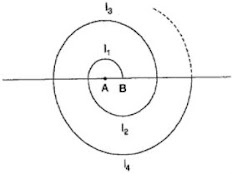19. 200 logs are stacked in the following manner: 20 logs in the bottom row, 19 in the next row, 18 in the row next to it and so on. In how many rows are the 200 logs placed and how many logs are in the top row?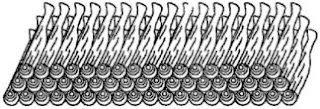20. In a potato race, a bucket is placed at the starting point, which is 5 m from the first potato and other potatoes are placed 3 m apart in a straight line. There are ten potatoes in the line.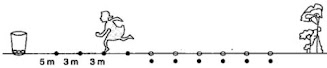A competitor starts from the bucket, picks up the nearest potato, runs back with it, drops it in the bucket, runs back to pick up the next potato, runs to the bucket to drop it in, and she continues in the same way until all the potatoes are in the bucket. What is the total distance the competitor has to run?
[Hint: to pick up the first potato and the second potato, the total distance (in metres) run by a competitor is 2×5+2×(5+3)]

Solution

o pick up first, second, third, ........ potaotes, distances covered are 10 m, 16m, 22m, ...... These form an A.P. with a = 10, d = 6.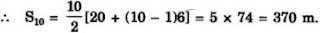#### Exercise 5.4

1. Which term of the AP: 121, 117, 113, . . ., is its first negative term? [Hint: Find n for an < 0]

2. The sum of the third and the seventh terms of an AP is 6 and their product is 8. Find the sum of first sixteen terms of the AP.

3. A ladder has rungs 25 cm apart. (see Fig. 5.7). The rungs decrease uniformly in length from 45 cm at the bottom to 25 cm at the top. If the top and the bottom rungs are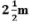apart, what is the length of the wood required for the rungs? [Hint: Number of rungs = -250/25 ].

4. The houses of a row are numbered consecutively from 1 to 49. Show that there is a value of x such that the sum of the numbers of the houses preceding the house numbered x is equal to the sum of the numbers of the houses following it. Find this value of x. [Hint :Sx – 1 = S49 – Sx ]

5. A small terrace at a football ground comprises of 15 steps each of which is 50 m long and built of solid concrete. Each step has a rise of 1 4 m and a tread of 1 2 m. (see Fig. 5.8). Calculate the total volume of concrete required to build the terrace. [Hint : Volume of concrete required to build the first step = ¼ ×1/2 ×50 m3.]